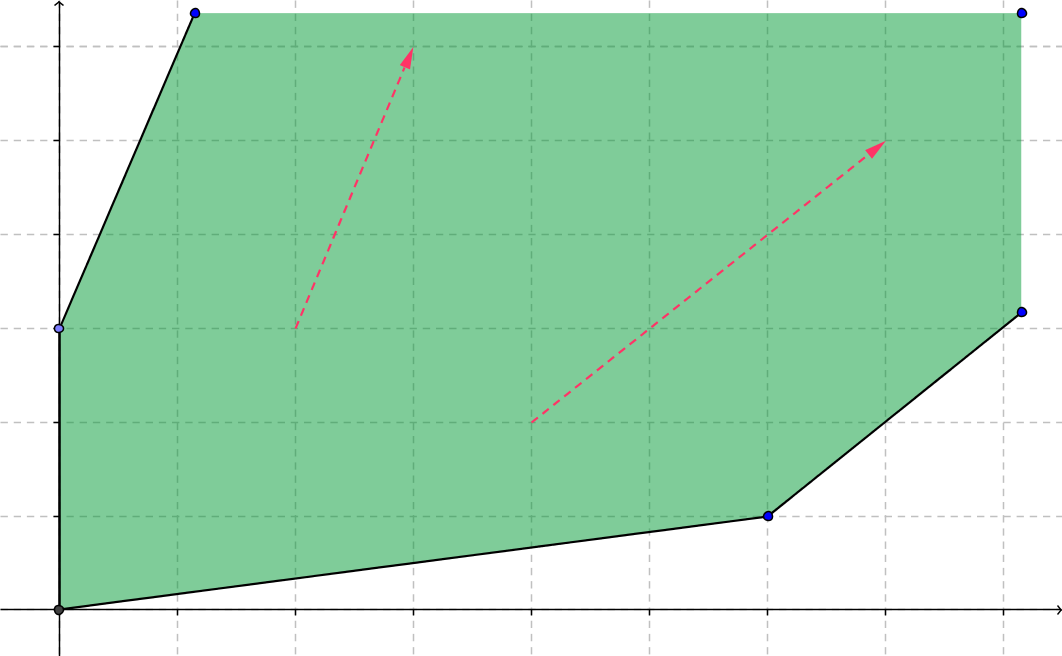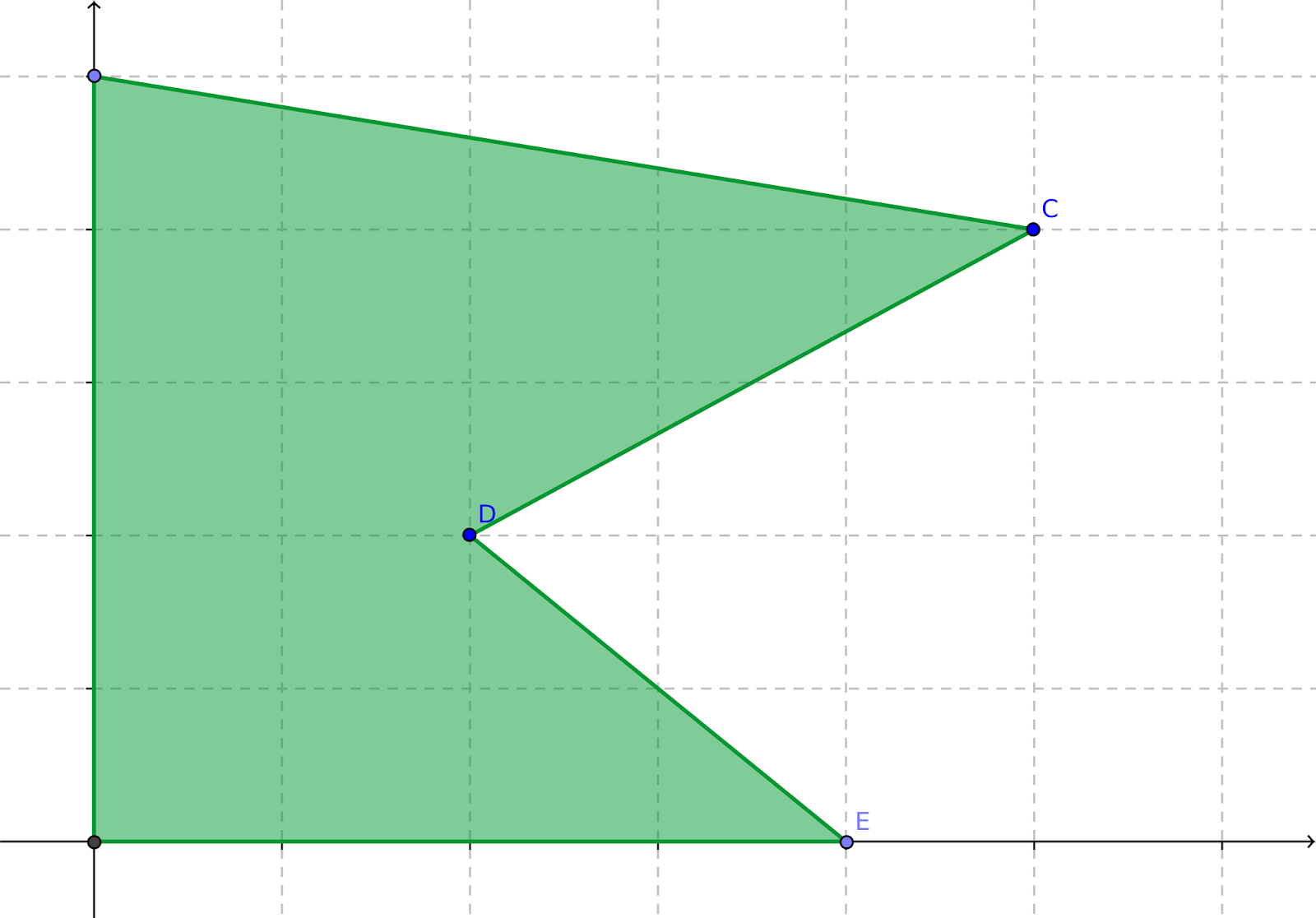## Friday, February 20, 2015

### The Geometry of a Linear Program

I frequently see questions on forums, in blog comments, or in my in-box that suggest the person asking the question is either unfamiliar with the geometry of a linear program, unsure of the significance of it, or just not connecting their question to the geometry. This post is just the starting point for addressing some of those questions. (I dislike reading or writing long posts, so I'm "chunking" my answer, and this is the first chunk.)

Ideally, the feasible region of a linear program (henceforth "LP") is a convex polytope, a geometric object that is convex, closed, bounded, and has a finite number of flat sides (intersecting at vertices or extreme points). Figure 1 shows a two dimensional example. The shaded area is the feasible region; the dots are the vertices.Figure 1: Polytope

Being closed and bounded (and therefore, in finite dimensions, compact) ensures, via the extreme value theorem, that any continuous objective function attains its maximum and minimum over the feasible region at one or more points. Of course, the objective function of a linear or quadratic program is continuous. For a linear objective function, we can be more specific: the optimum will be attained at one or more extreme points. So we only need to check the vertices, and this in essence is what the famed simplex algorithm does.

To see why that is important, consider the following candidate for world's most trivial LP:$\begin{array}{lrcl} \textrm{minimize} & x\\ \textrm{subject to} & x & \ge & 0. \end{array}$ Its solution is, shockingly, $x=0$. Now suppose we try to use a strict inequality in the lone constraint:$\begin{array}{lrcl} \textrm{minimize} & x\\ \textrm{subject to} & x & \gt & 0. \end{array}$The lower bound of the objective function is again 0, but it is never attained, since $x=0$ is not a feasible solution. This is a consequence of the feasible region not being closed. Technically, while the objective function ($f(x)=x$) has an infimum over the feasible region, it does not have a minimum. While trivial, this is a useful example of why we never use strict inequalities (>, <) in a mathematical program.

One step more general than a polytope is a polyhedron, which retains all the characteristics of a polytope except boundedness. Figure 2 illustrates a polyhedron.Figure 2: Polyhedron
Again, we have a finite number of flat sides (the dark line segments) intersecting in vertices (the dots), and again the region is convex and closed. The arrows indicate recession directions, directions in which we can continue forever without leaving the polyhedron. (Any direction between those two is also a recession direction.) The presence of recession directions makes the question of whether the (continuous) objective function attains a maximum or minimum a bit trickier:
• if the objective function degrades (gets bigger if minimizing, smaller if maximizing) along every recession direction, the optimal value of the objective function will be attained at one or more extreme points;
• if the objective function improves (gets smaller if minimizing, bigger if maximizing) along any recession direction, the problem is unbounded (the objective function does not have a maximum or minimum, whichever one you were hunting), which likely means you omitted or screwed up a constraint; and
• if the objective function is constant along at least one recession direction
and does not improve along any of them, the objective function achieves its optimum at one or more extreme points and along every ray starting from one of those extreme points and pointing in a recession direction where the objective stays constant.
Finally, a word about the role of convexity. Consider the feasible region in Figure 3, which is a polytope but is not convex.Figure 3: Nonconvex polytope
Suppose that we want to find the point furthest to the right (maximize $x$, assuming that the horizontal axis represents $x$ and the vertical axis represents a second variable $y$). The optimal solution is clearly at point C. Suppose further that we currently find ourselves at point E, which is a local optimum but not a global one. For any algorithm to get from E to C, it has to move in a direction that decreases $x$ (making the objective function worse than at E, at least in the short run). That is a consequence of the fact that any line segment from E in the direction of C initially leaves the feasible region, which in turn follows from the lack of convexity. Most optimization algorithms (including the simplex algorithm) are not "smart" enough to do this; they tend to be myopic, moving only in directions that immediately improve the objective function. LPs are as tractable as they are in large part because their feasible regions are guaranteed to be convex.

1.I think you may be having an issue with your LaTeX renderer (MathJax?). I'm seeing things like $x$ in the post.
1.